# RS Aggarwal Solutions for Class 10 Maths Chapter 2 Polynomials Exercise 2C

RS Aggarwal Solutions for Class 10 Maths Chapter 2 Polynomials, has solutions for all the exercise questions. Exercise 2C is mainly based on polynomials, types, zeros of a polynomial and relationship between zeros and coefficients of a quadratic Polynomial. Now download the RS Aggarwal Solutions of Class 10 Maths and speed up your exam preparation.

### Download PDF of RS Aggarwal Solutions for Class 10 Maths Chapter 2 Polynomials Exercise 2C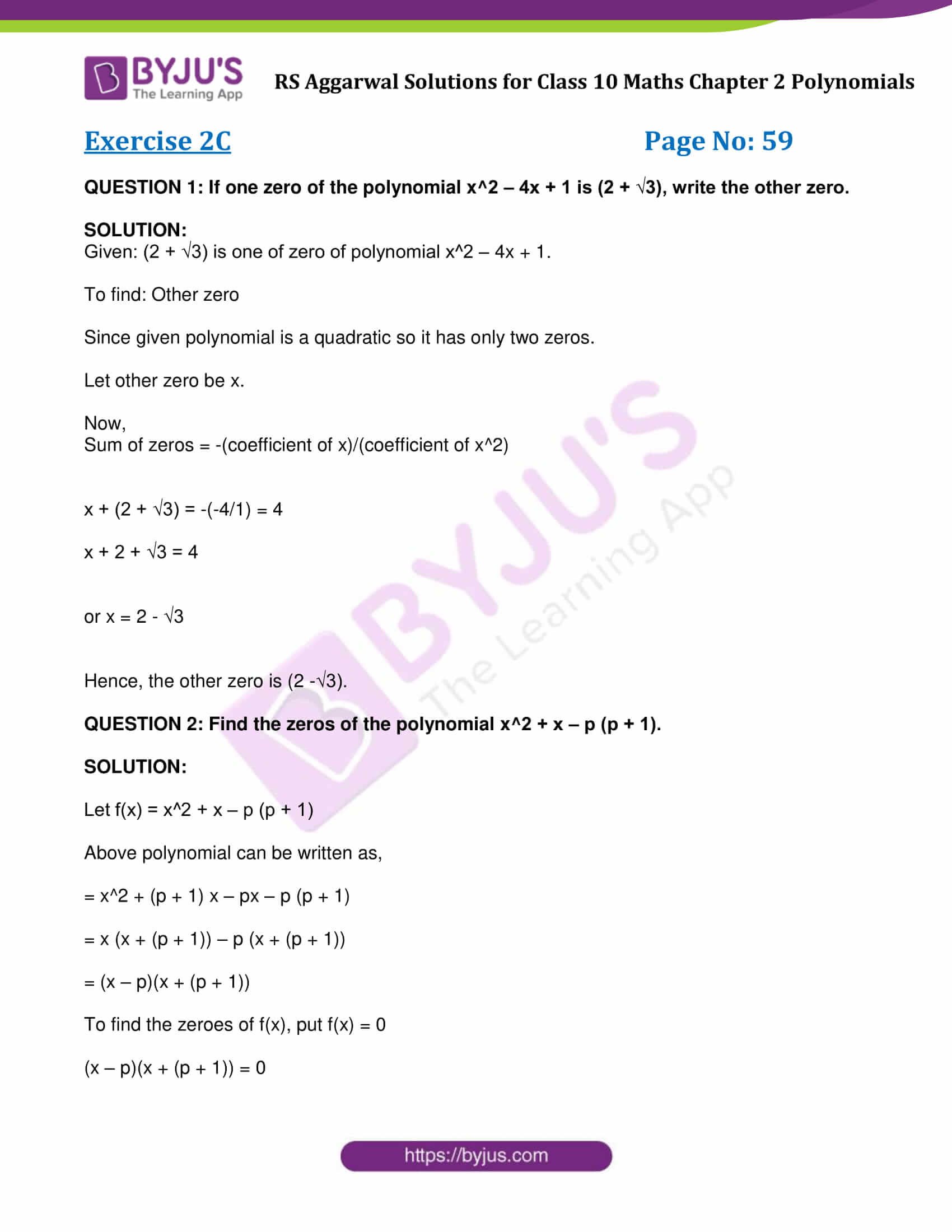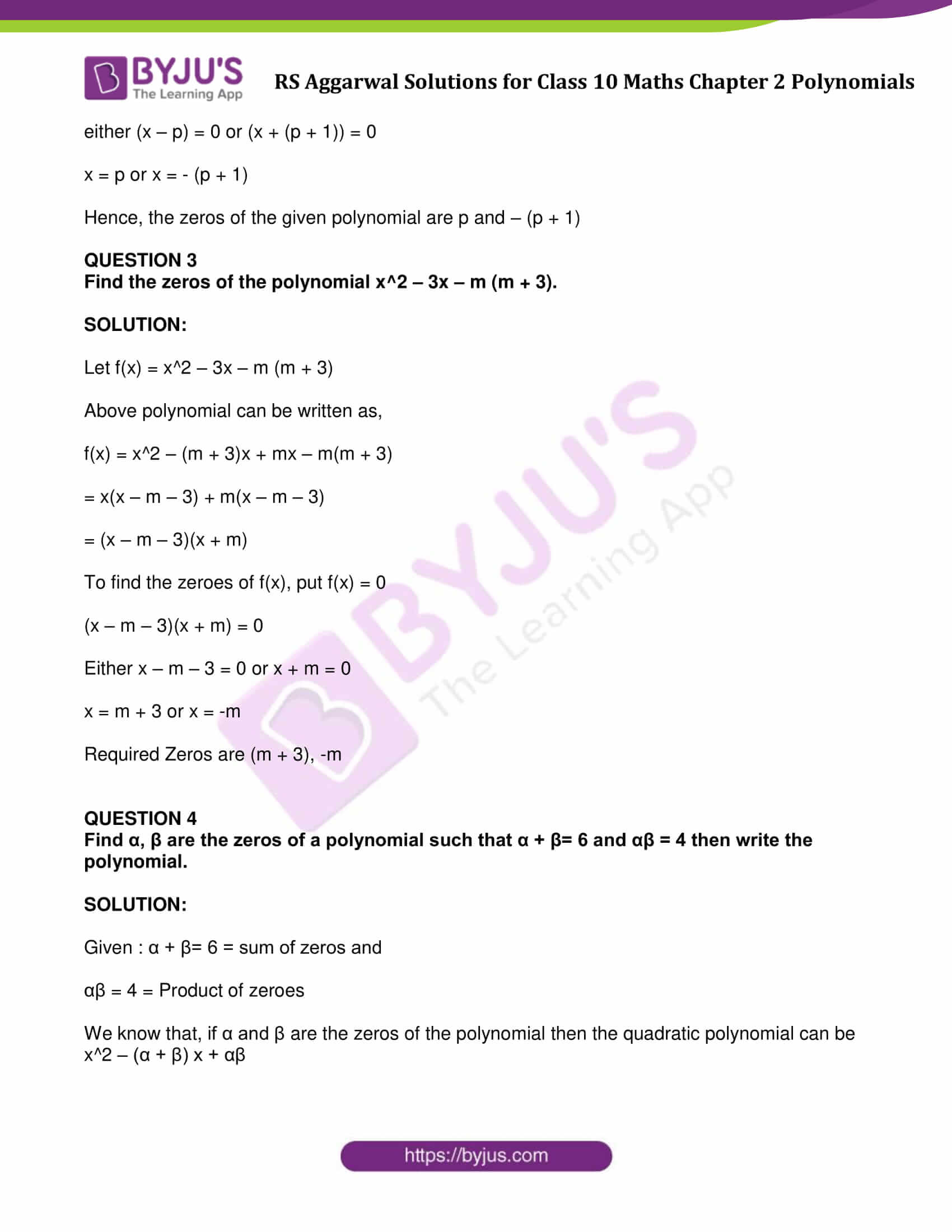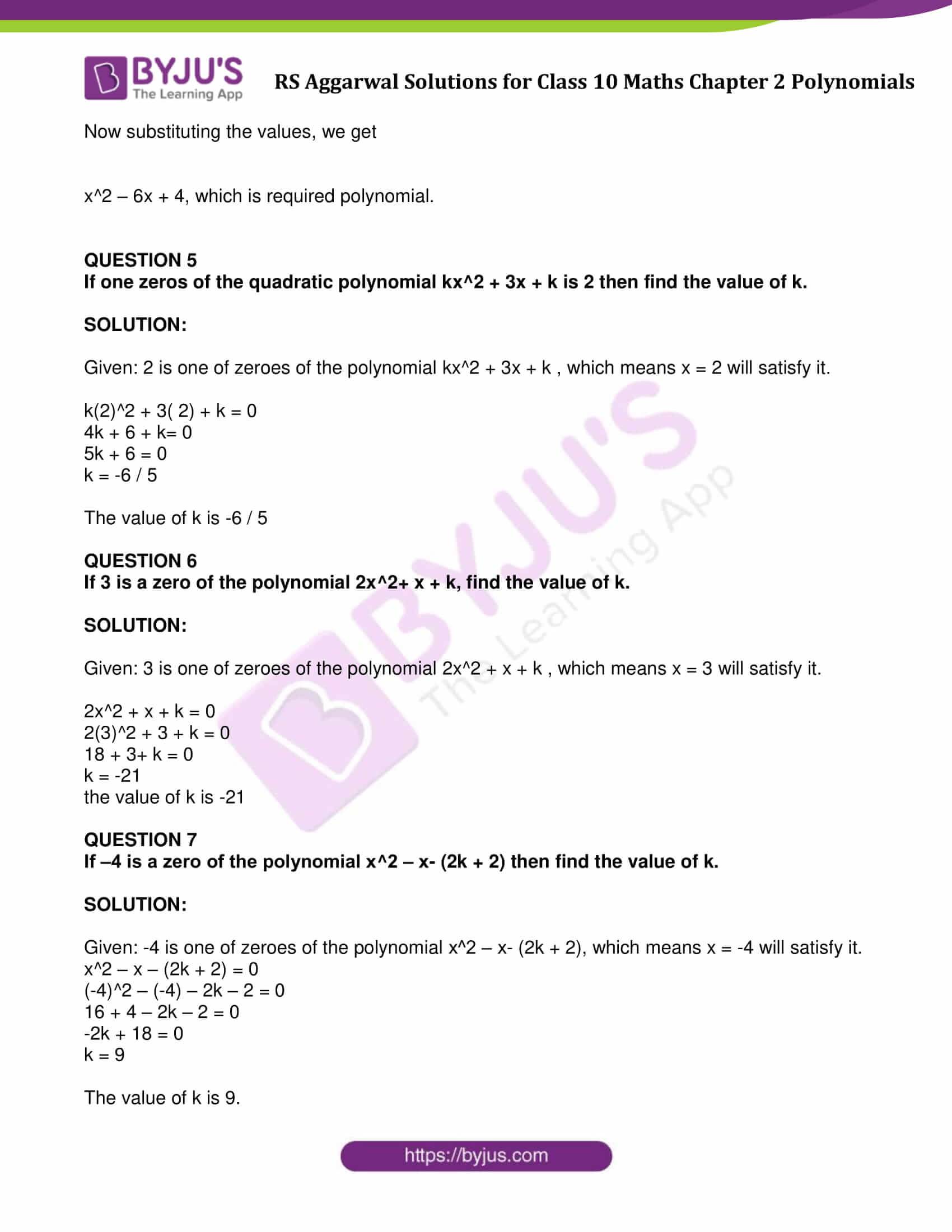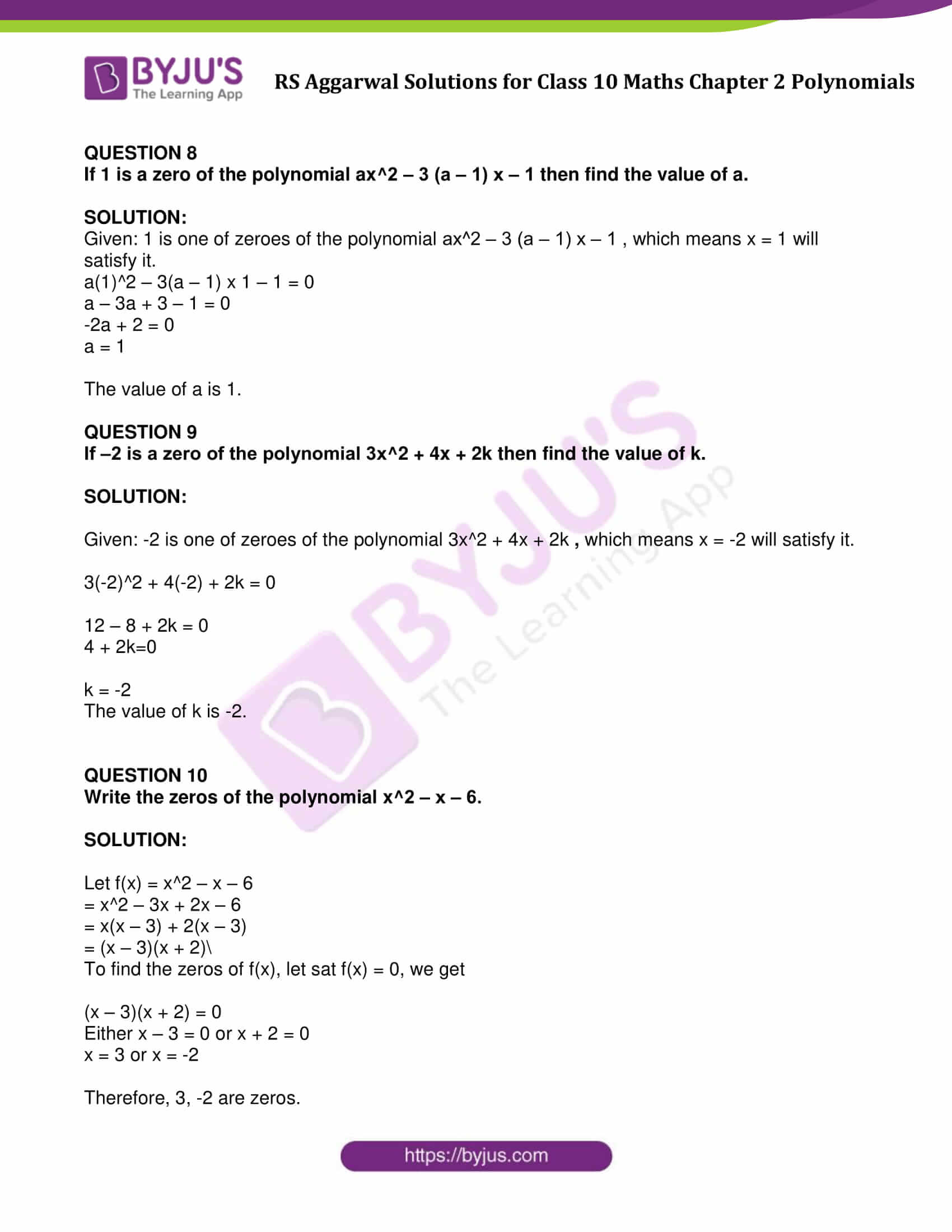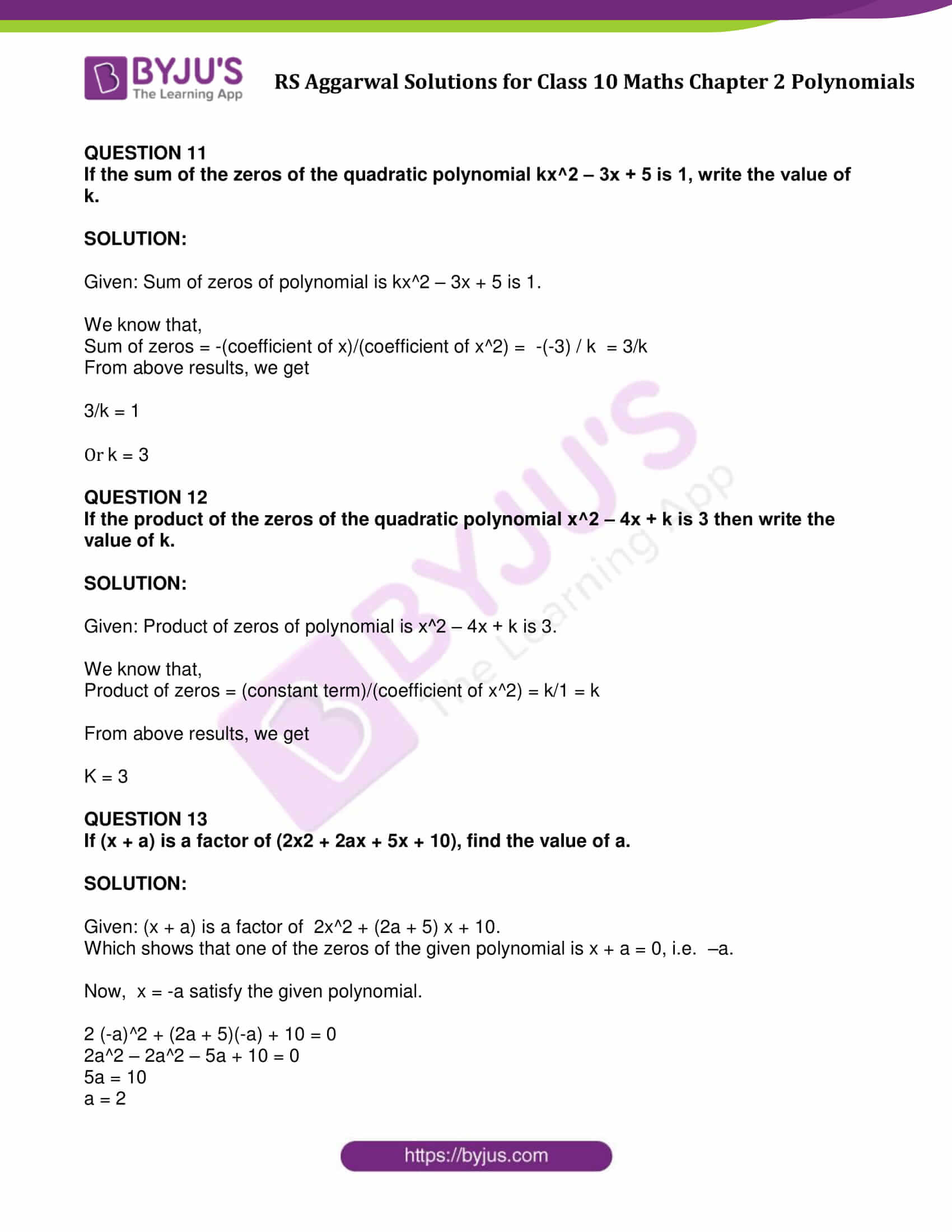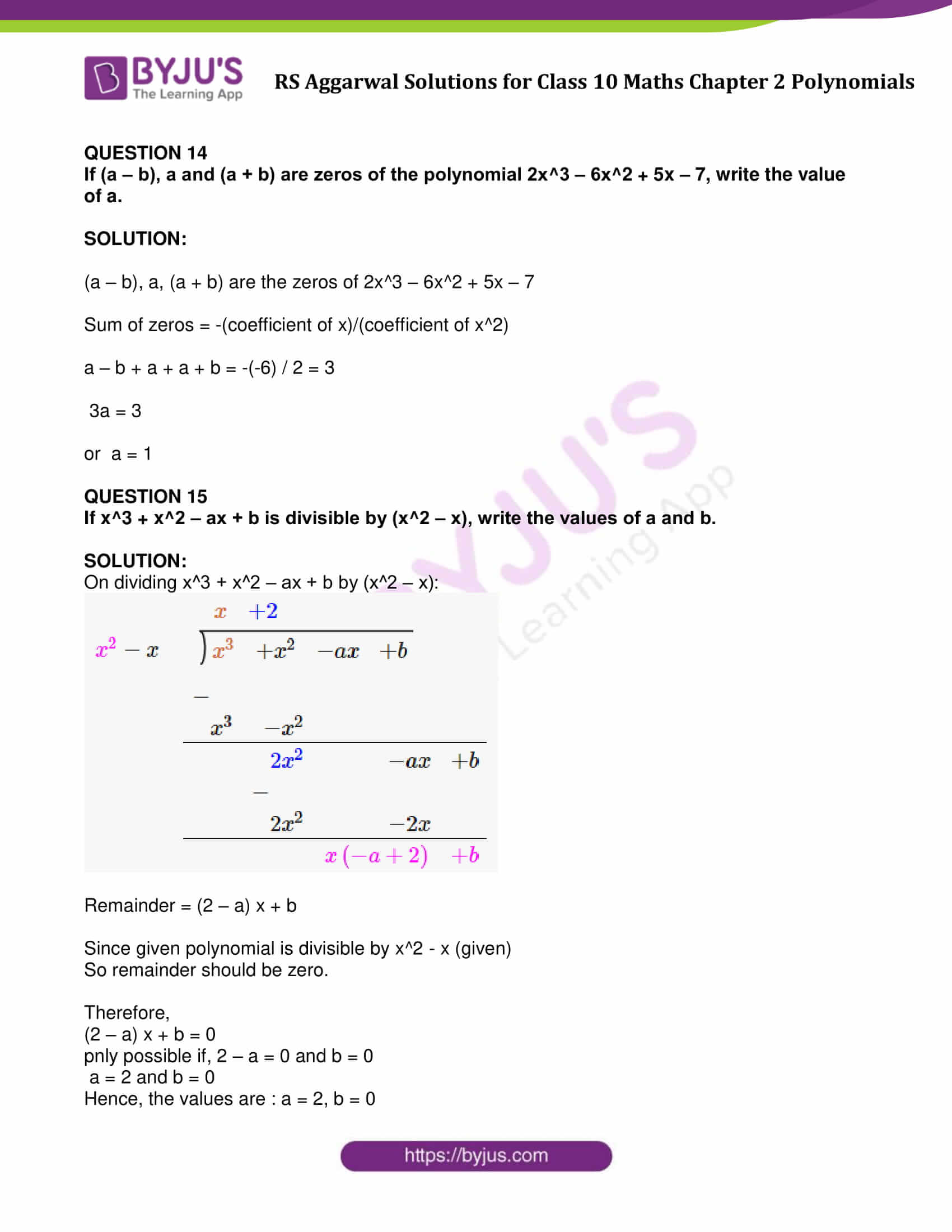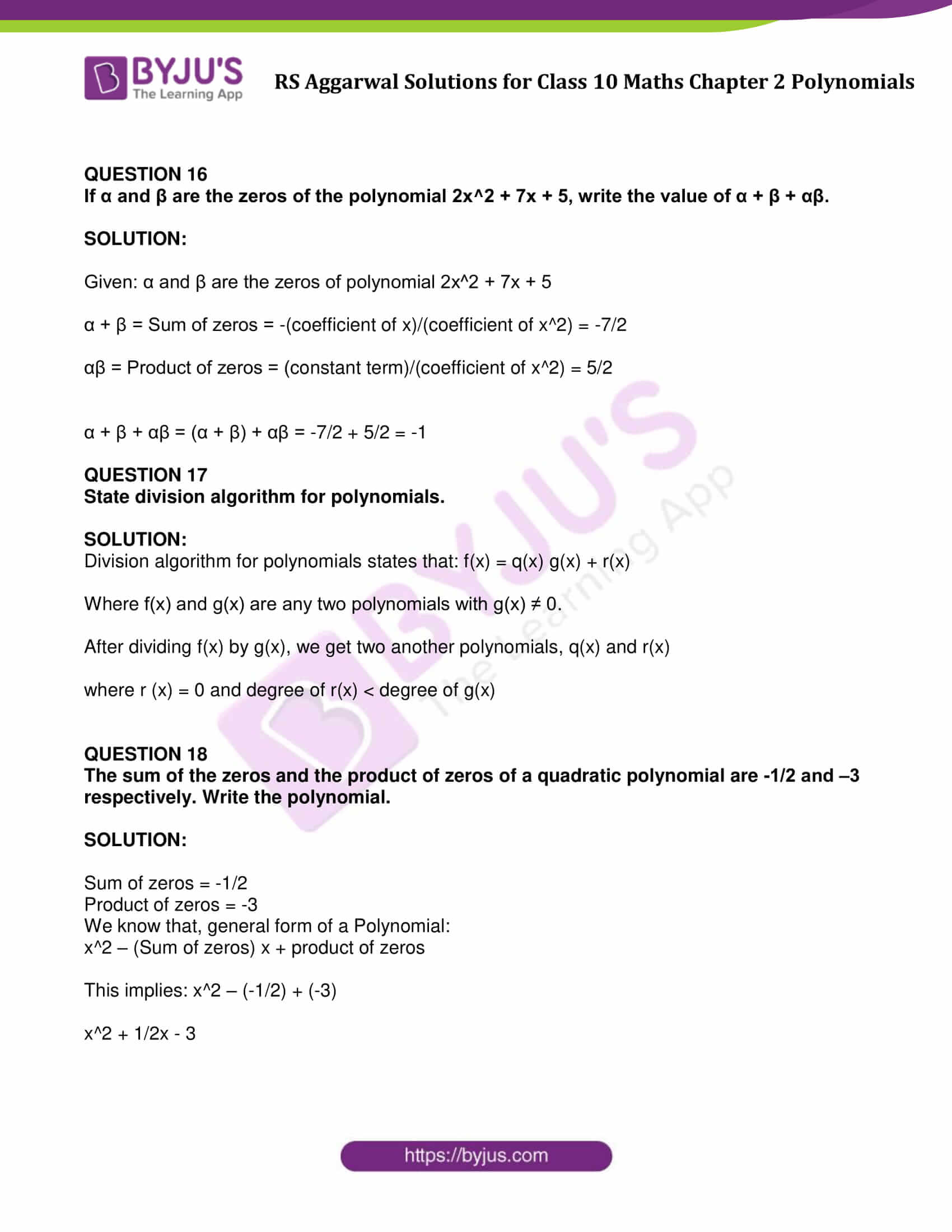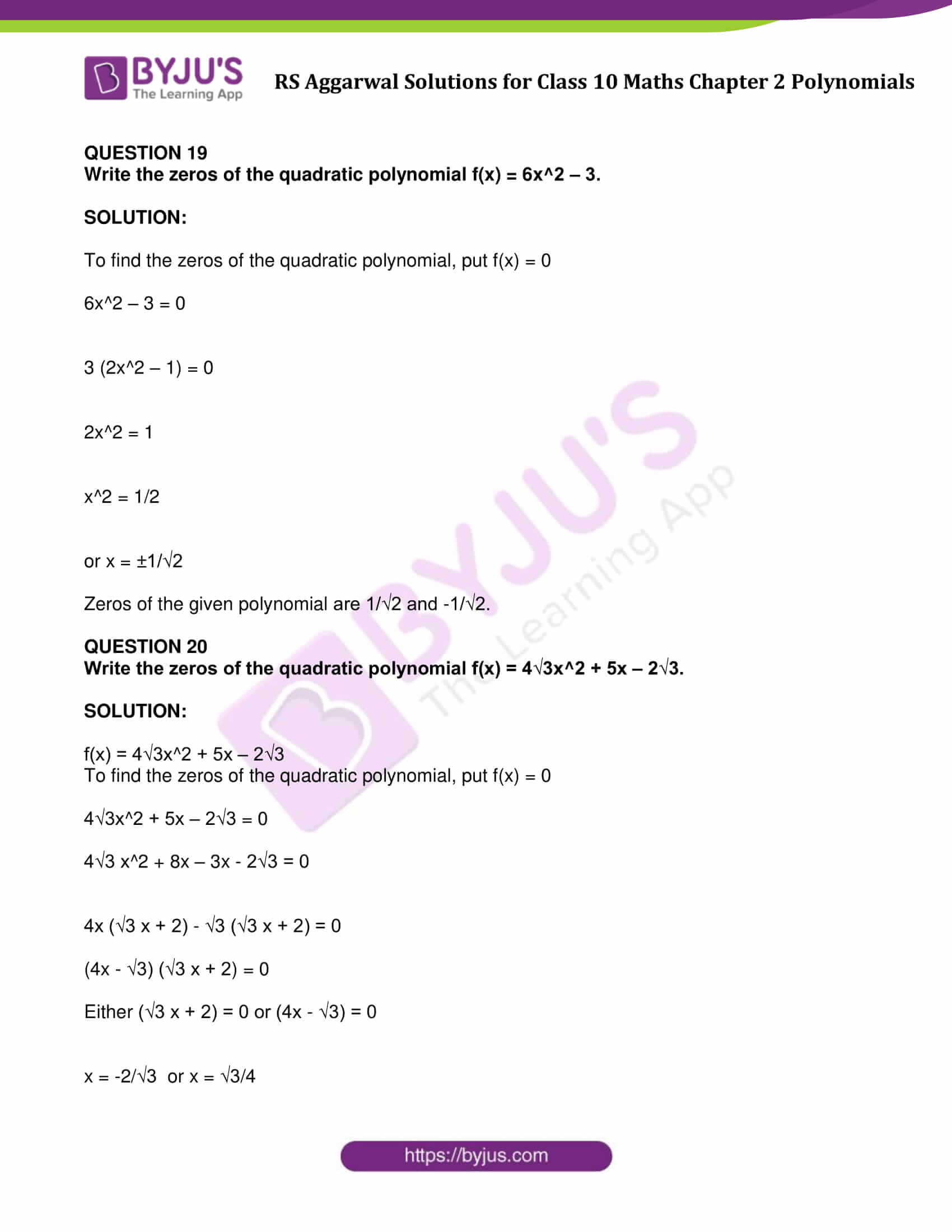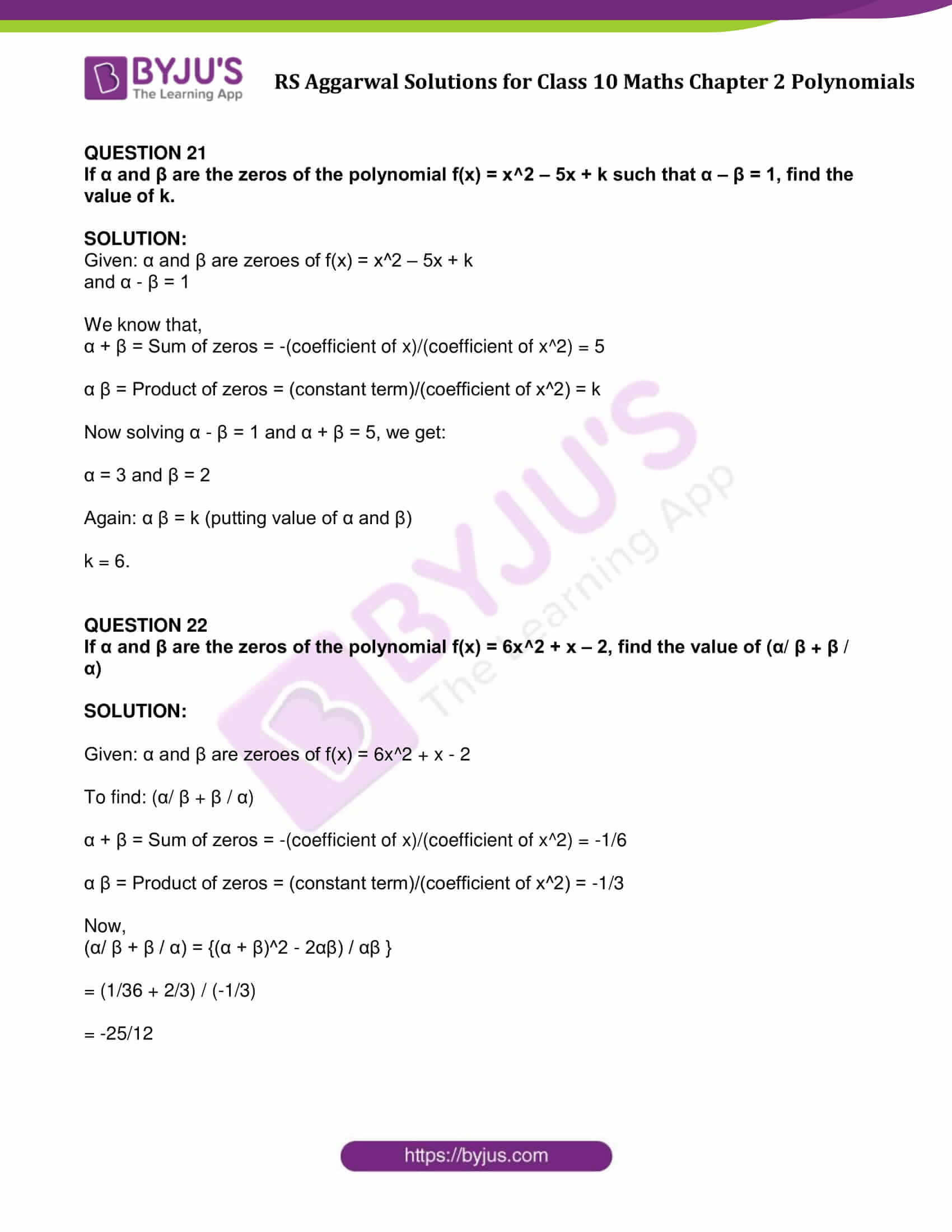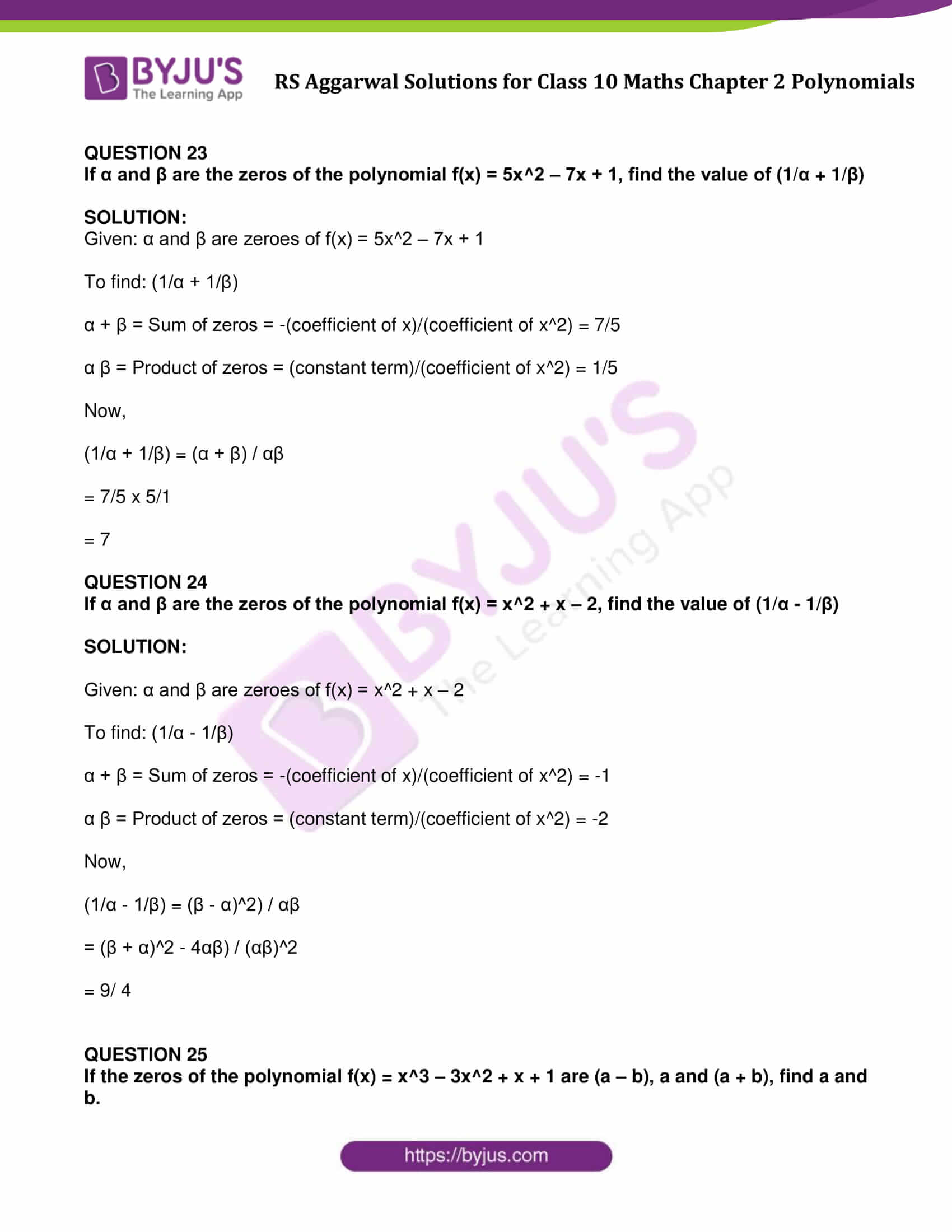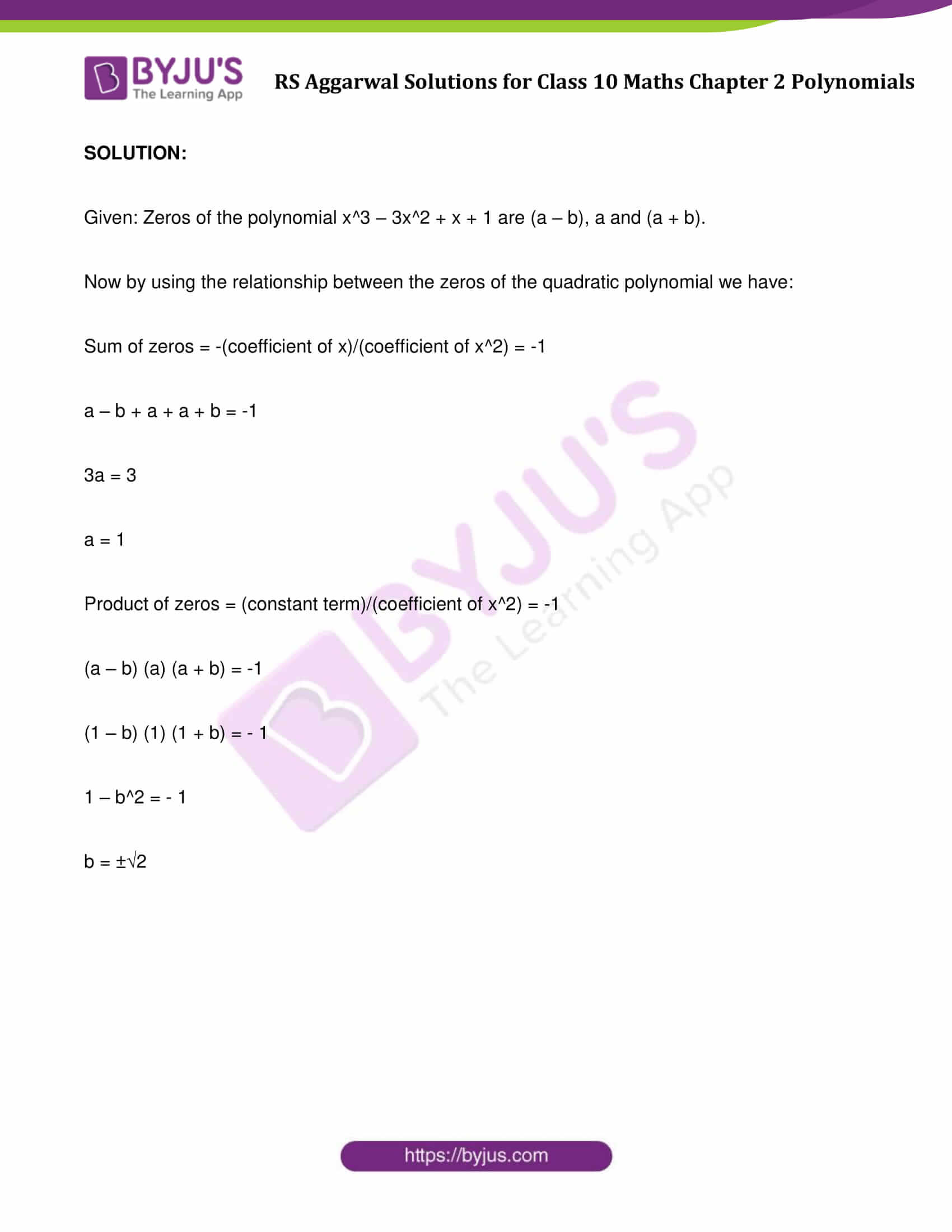### Access other exercise solutions of Class 10 Maths Chapter 2 Polynomials

Exercise 2A Solutions: 21 Questions (Short Answers)

Exercise 2B Solutions: 19 Questions (Short Answers)

## Exercise 2C Page No: 59

QUESTION 1: If one zero of the polynomial x^2 ‒ 4x + 1 is (2 + √3), write the other zero.

SOLUTION:

Given: (2 + √3) is one of zero of polynomial x^2 ‒ 4x + 1.

To find: Other zero

Since given polynomial is a quadratic so it has only two zeros.

Let other zero be x.

Now,

Sum of zeros = -(coefficient of x)/(coefficient of x^2)

x + (2 + √3) = -(-4/1) = 4

x + 2 + √3 = 4

or x = 2 – √3

Hence, the other zero is (2 -√3).

QUESTION 2: Find the zeros of the polynomial x^2 + x ‒ p (p + 1).

SOLUTION:

Let f(x) = x^2 + x ‒ p (p + 1)

Above polynomial can be written as,

= x^2 + (p + 1) x – px – p (p + 1)

= x (x + (p + 1)) – p (x + (p + 1))

= (x – p)(x + (p + 1))

To find the zeroes of f(x), put f(x) = 0

(x – p)(x + (p + 1)) = 0

either (x – p) = 0 or (x + (p + 1)) = 0

x = p or x = – (p + 1)

Hence, the zeros of the given polynomial are p and – (p + 1)

QUESTION 3

Find the zeros of the polynomial x^2 – 3x – m (m + 3).

SOLUTION:

Let f(x) = x^2 – 3x – m (m + 3)

Above polynomial can be written as,

f(x) = x^2 – (m + 3)x + mx – m(m + 3)

= x(x – m – 3) + m(x – m – 3)

= (x – m – 3)(x + m)

To find the zeroes of f(x), put f(x) = 0

(x – m – 3)(x + m) = 0

Either x – m – 3 = 0 or x + m = 0

x = m + 3 or x = -m

Required Zeros are (m + 3), -m

QUESTION 4

Find α, β are the zeros of a polynomial such that α + β= 6 and αβ = 4 then write the polynomial.

SOLUTION:

Given : α + β= 6 = sum of zeros and

αβ = 4 = Product of zeroes

We know that, if α and β are the zeros of the polynomial then the quadratic polynomial can be x^2 – (α + β) x + αβ

Now substituting the values, we get

x^2 – 6x + 4, which is required polynomial.

QUESTION 5

If one zeros of the quadratic polynomial kx^2 + 3x + k is 2 then find the value of k.

SOLUTION:

Given: 2 is one of zeroes of the polynomial kx^2 + 3x + k , which means x = 2 will satisfy it.

k(2)^2 + 3( 2) + k = 0

4k + 6 + k= 0

5k + 6 = 0

k = -6 / 5

The value of k is -6 / 5

QUESTION 6

If 3 is a zero of the polynomial 2x^2+ x + k, find the value of k.

SOLUTION:

Given: 3 is one of zeroes of the polynomial 2x^2 + x + k , which means x = 3 will satisfy it.

2x^2 + x + k = 0

2(3)^2 + 3 + k = 0

18 + 3+ k = 0

k = -21

the value of k is -21

QUESTION 7

If ‒4 is a zero of the polynomial x^2 ‒ x- (2k + 2) then find the value of k.

SOLUTION:

Given: -4 is one of zeroes of the polynomial x^2 ‒ x- (2k + 2), which means x = -4 will satisfy it.

x^2 – x – (2k + 2) = 0

(-4)^2 – (-4) – 2k – 2 = 0

16 + 4 – 2k – 2 = 0

-2k + 18 = 0

k = 9

The value of k is 9.

QUESTION 8

If 1 is a zero of the polynomial ax^2 ‒ 3 (a ‒ 1) x ‒ 1 then find the value of a.

SOLUTION:

Given: 1 is one of zeroes of the polynomial ax^2 ‒ 3 (a ‒ 1) x ‒ 1 , which means x = 1 will satisfy it.

a(1)^2 – 3(a – 1) x 1 – 1 = 0

a – 3a + 3 – 1 = 0

-2a + 2 = 0

a = 1

The value of a is 1.

QUESTION 9

If ‒2 is a zero of the polynomial 3x^2 + 4x + 2k then find the value of k.

SOLUTION:

Given: -2 is one of zeroes of the polynomial 3x^2 + 4x + 2k , which means x = -2 will satisfy it.

3(-2)^2 + 4(-2) + 2k = 0

12 – 8 + 2k = 0

4 + 2k=0

k = -2

The value of k is -2.

QUESTION 10

Write the zeros of the polynomial x^2 ‒ x ‒ 6.

SOLUTION:

Let f(x) = x^2 – x – 6

= x^2 – 3x + 2x – 6

= x(x – 3) + 2(x – 3)

= (x – 3)(x + 2)\

To find the zeros of f(x), let sat f(x) = 0, we get

(x – 3)(x + 2) = 0

Either x – 3 = 0 or x + 2 = 0

x = 3 or x = -2

Therefore, 3, -2 are zeros.

QUESTION 11

If the sum of the zeros of the quadratic polynomial kx^2 ‒ 3x + 5 is 1, write the value of k.

SOLUTION:

Given: Sum of zeros of polynomial is kx^2 – 3x + 5 is 1.

We know that,

Sum of zeros = -(coefficient of x)/(coefficient of x^2) = -(-3) / k = 3/k

From above results, we get

3/k = 1

Or k = 3

QUESTION 12

If the product of the zeros of the quadratic polynomial x^2 ‒ 4x + k is 3 then write the value of k.

SOLUTION:

Given: Product of zeros of polynomial is x^2 ‒ 4x + k is 3.

We know that,

Product of zeros = (constant term)/(coefficient of x^2) = k/1 = k

From above results, we get

K = 3

QUESTION 13

If (x + a) is a factor of (2×2 + 2ax + 5x + 10), find the value of a.

SOLUTION:

Given: (x + a) is a factor of 2x^2 + (2a + 5) x + 10.

Which shows that one of the zeros of the given polynomial is x + a = 0, i.e. –a.

Now, x = -a satisfy the given polynomial.

2 (-a)^2 + (2a + 5)(-a) + 10 = 0

2a^2 – 2a^2 – 5a + 10 = 0

5a = 10

a = 2

QUESTION 14

If (a ‒ b), a and (a + b) are zeros of the polynomial 2x^3 ‒ 6x^2 + 5x – 7, write the value of a.

SOLUTION:

(a – b), a, (a + b) are the zeros of 2x^3 – 6x^2 + 5x – 7

Sum of zeros = -(coefficient of x)/(coefficient of x^2)

a – b + a + a + b = -(-6) / 2 = 3

3a = 3

or a = 1

QUESTION 15

If x^3 + x^2 ‒ ax + b is divisible by (x^2 ‒ x), write the values of a and b.

SOLUTION:

On dividing x^3 + x^2 ‒ ax + b by (x^2 ‒ x):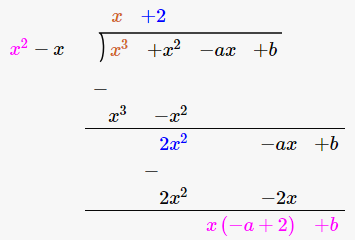Remainder = (2 – a) x + b

Since given polynomial is divisible by x^2 – x (given)

So remainder should be zero.

Therefore,

(2 – a) x + b = 0

pnly possible if, 2 – a = 0 and b = 0

a = 2 and b = 0

Hence, the values are : a = 2, b = 0

QUESTION 16

If α and β are the zeros of the polynomial 2x^2 + 7x + 5, write the value of α + β + αβ.

SOLUTION:

Given: α and β are the zeros of polynomial 2x^2 + 7x + 5

α + β = Sum of zeros = -(coefficient of x)/(coefficient of x^2) = -7/2

αβ = Product of zeros = (constant term)/(coefficient of x^2) = 5/2

α + β + αβ = (α + β) + αβ = -7/2 + 5/2 = -1

QUESTION 17

State division algorithm for polynomials.

SOLUTION:

Division algorithm for polynomials states that: f(x) = q(x) g(x) + r(x)

Where f(x) and g(x) are any two polynomials with g(x) ≠ 0.

After dividing f(x) by g(x), we get two another polynomials, q(x) and r(x)

where r (x) = 0 and degree of r(x) < degree of g(x)

QUESTION 18

The sum of the zeros and the product of zeros of a quadratic polynomial are -1/2 and ‒3 respectively. Write the polynomial.

SOLUTION:

Sum of zeros = -1/2

Product of zeros = -3

We know that, general form of a Polynomial:

x^2 – (Sum of zeros) x + product of zeros

This implies: x^2 – (-1/2) + (-3)

x^2 + 1/2x – 3

QUESTION 19

Write the zeros of the quadratic polynomial f(x) = 6x^2 ‒ 3.

SOLUTION:

To find the zeros of the quadratic polynomial, put f(x) = 0

6x^2 – 3 = 0

3 (2x^2 – 1) = 0

2x^2 = 1

x^2 = 1/2

or x = ±1/√2

Zeros of the given polynomial are 1/√2 and -1/√2.

QUESTION 20

Write the zeros of the quadratic polynomial f(x) = 4√3x^2 + 5x ‒ 2√3.

SOLUTION:

f(x) = 4√3x^2 + 5x ‒ 2√3

To find the zeros of the quadratic polynomial, put f(x) = 0

4√3x^2 + 5x ‒ 2√3 = 0

4√3 x^2 + 8x – 3x – 2√3 = 0

4x (√3 x + 2) – √3 (√3 x + 2) = 0

(4x – √3) (√3 x + 2) = 0

Either (√3 x + 2) = 0 or (4x – √3) = 0

x = -2/√3 or x = √3/4

QUESTION 21

If α and β are the zeros of the polynomial f(x) = x^2 ‒ 5x + k such that α ‒ β = 1, find the value of k.

SOLUTION:

Given: α and β are zeroes of f(x) = x^2 ‒ 5x + k

and α – β = 1

We know that,

α + β = Sum of zeros = -(coefficient of x)/(coefficient of x^2) = 5

α β = Product of zeros = (constant term)/(coefficient of x^2) = k

Now solving α – β = 1 and α + β = 5, we get:

α = 3 and β = 2

Again: α β = k (putting value of α and β)

k = 6.

QUESTION 22

If α and β are the zeros of the polynomial f(x) = 6x^2 + x ‒ 2, find the value of (α/ β + β / α)

SOLUTION:

Given: α and β are zeroes of f(x) = 6x^2 + x – 2

To find: (α/ β + β / α)

α + β = Sum of zeros = -(coefficient of x)/(coefficient of x^2) = -1/6

α β = Product of zeros = (constant term)/(coefficient of x^2) = -1/3

Now,

(α/ β + β / α) = {(α + β)^2 – 2αβ) / αβ }

= (1/36 + 2/3) / (-1/3)

= -25/12

QUESTION 23

If α and β are the zeros of the polynomial f(x) = 5x^2 ‒ 7x + 1, find the value of (1/α + 1/β)

SOLUTION:

Given: α and β are zeroes of f(x) = 5x^2 ‒ 7x + 1

To find: (1/α + 1/β)

α + β = Sum of zeros = -(coefficient of x)/(coefficient of x^2) = 7/5

α β = Product of zeros = (constant term)/(coefficient of x^2) = 1/5

Now,

(1/α + 1/β) = (α + β) / αβ

= 7/5 x 5/1

= 7

QUESTION 24

If α and β are the zeros of the polynomial f(x) = x^2 + x – 2, find the value of (1/α – 1/β)

SOLUTION:

Given: α and β are zeroes of f(x) = x^2 + x – 2

To find: (1/α – 1/β)

α + β = Sum of zeros = -(coefficient of x)/(coefficient of x^2) = -1

α β = Product of zeros = (constant term)/(coefficient of x^2) = -2

Now,

(1/α – 1/β) = (β – α)^2) / αβ

= (β + α)^2 – 4αβ) / (αβ)^2

= 9/ 4

QUESTION 25

If the zeros of the polynomial f(x) = x^3 – 3x^2 + x + 1 are (a – b), a and (a + b), find a and b.

SOLUTION:

Given: Zeros of the polynomial x^3 – 3x^2 + x + 1 are (a – b), a and (a + b).

Now by using the relationship between the zeros of the quadratic polynomial we have:

Sum of zeros = -(coefficient of x)/(coefficient of x^2) = -1

a – b + a + a + b = -1

3a = 3

a = 1

Product of zeros = (constant term)/(coefficient of x^2) = -1

(a – b) (a) (a + b) = -1

(1 – b) (1) (1 + b) = – 1

1 – b^2 = – 1

b = ±√2

## RS Aggarwal Solutions for Class 10 Maths Chapter 2 Polynomials Exercise 2C

Class 10 Maths Chapter 2 Polynomials Exercise 2C is based on the following topics and subtopics:

• Value of a Polynomials at a given point
• Zeros of a Polynomials
• Relation between the zeros and coefficients of a quadratic Polynomial